# RD Sharma Class 8 Solutions Chapter 1 Rational Numbers Ex 1.2

In this chapter, we provide RD Sharma Class 8 Solutions Chapter 1 Rational Numbers Ex 1.2 for English medium students, Which will very helpful for every student in their exams. Students can download the latest RD Sharma Class 8 Solutions Chapter 1 Rational Numbers Ex 1.2 Maths pdf, free RD Sharma Class 8 Solutions Chapter 1 Rational Numbers Ex 1.2 Maths book pdf download. Now you will get step by step solution to each question.

### RD Sharma Solutions for Class 8 Chapter 1 Rational Numbers Ex 1.2 Download PDF

Question 1.
Verify commutativity of addition of rational numbers for each of the following pairs of rational numbers :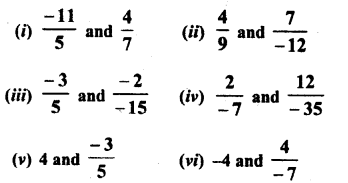Solution: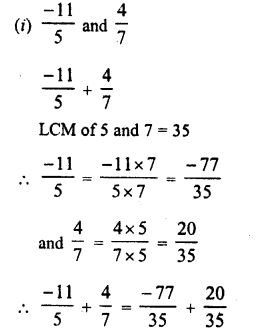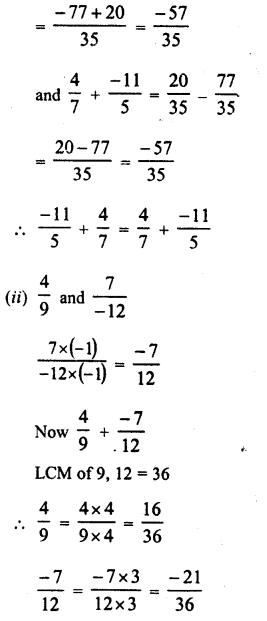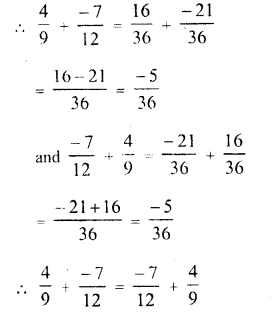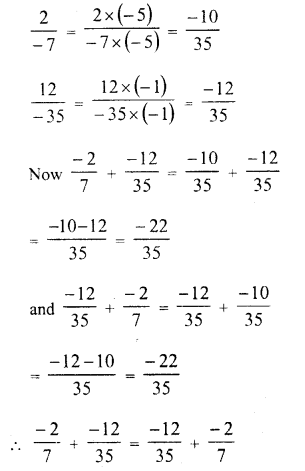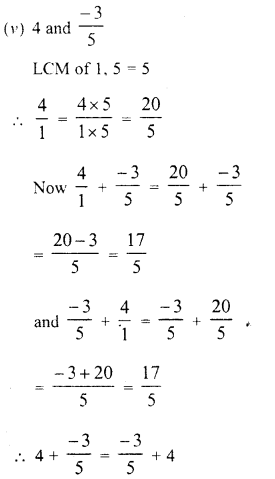Question 2.
Verify associativity of addition of rational numbers i.e., (A: + y) + z = x + (y + z), when :Solution: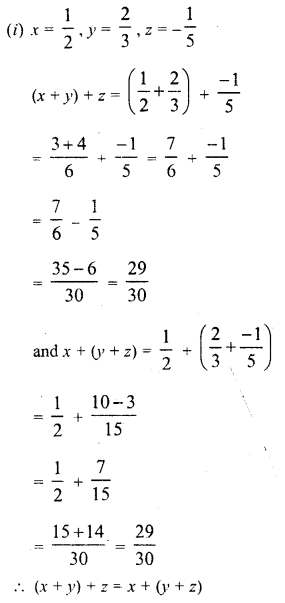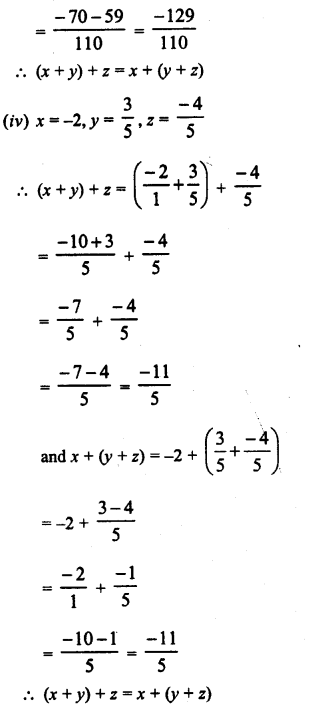Question 3.
Write the additive inverse of each of the following rational numbers :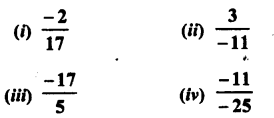Solution:Question 4.
Write the negative (additive inverse) of each of the following :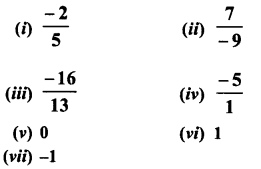Solution: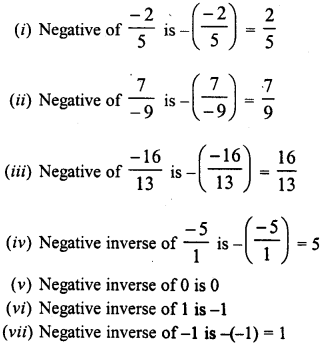Question 5.
Using commutativity and associativity of addition of rational numbers, express ‘iach of the following as a rational number :Solution: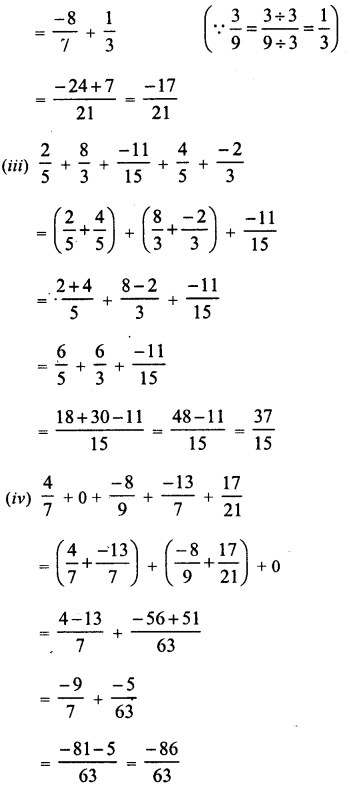Question 6.
Re-arrange suitably and find the sum in each of the following :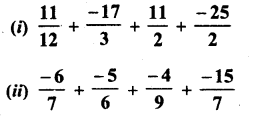Solution:All Chapter RD Sharma Solutions For Class 8 Maths

—————————————————————————–

All Subject NCERT Exemplar Problems Solutions For Class 8

All Subject NCERT Solutions For Class 8

*************************************************

I think you got complete solutions for this chapter. If You have any queries regarding this chapter, please comment on the below section our subject teacher will answer you. We tried our best to give complete solutions so you got good marks in your exam.

If these solutions have helped you, you can also share rdsharmasolutions.in to your friends.